Get inspired by the success stories of our students in IIT JAM MS, ISI  MStat, CMI MSc DS.  Learn More

# ISI MStat PSB 2007 Problem 1 | Determinant and Eigenvalues of a matrixThis is a very beautiful sample problem from ISI MStat PSB 2007 Problem 1 based on Determinant and Eigen values and Eigen vectors . Let's give it a try !!

## Problem- ISI MStat PSB 2007 Problem 1

Let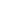be a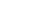matrix with real entries such that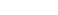Find the determinant of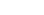where I denotes the identity matrix.

Determinant

Eigen Values

Eigen Vectors

## Solution :

Let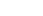be two eigen values of A then ,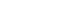.

Now it's given that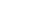, so we have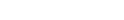. You may verify it ! (Hint : use the theorem that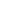is a eigen value of matrix B and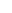is it's corresponding eigen value then we can write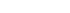or , use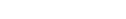).

Hence we have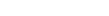.

Now , eigen values of Identity matrix I are 1 . So, we can write for eigen valueof (A+I) ,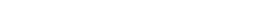.

Thus we get that both the eigen values of (A+1) are 1 . Again we know that determinant of a matrix is product of it's eigen values .

So, we have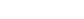.

Do you think this solution is correct ?

If yes , then you are absolutely wrong . The mistake is in assuming A and I has same eigen vectors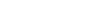Correct Solution

We have shown in first part of wrong solution that A has eigen values 0 . Hence the characteristic polynomial of A can be written as ,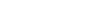.

Now taking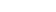we get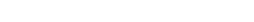.

## Food For Thought

If we are given that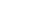for positive integer n , instead ofthen find the same .

## Subscribe to Cheenta at Youtube

This is a very beautiful sample problem from ISI MStat PSB 2007 Problem 1 based on Determinant and Eigen values and Eigen vectors . Let's give it a try !!

## Problem- ISI MStat PSB 2007 Problem 1

Letbe amatrix with real entries such thatFind the determinant ofwhere I denotes the identity matrix.

Determinant

Eigen Values

Eigen Vectors

## Solution :

Letbe two eigen values of A then ,.

Now it's given that, so we have. You may verify it ! (Hint : use the theorem thatis a eigen value of matrix B andis it's corresponding eigen value then we can writeor , use).

Hence we have.

Now , eigen values of Identity matrix I are 1 . So, we can write for eigen valueof (A+I) ,.

Thus we get that both the eigen values of (A+1) are 1 . Again we know that determinant of a matrix is product of it's eigen values .

So, we have.

Do you think this solution is correct ?

If yes , then you are absolutely wrong . The mistake is in assuming A and I has same eigen vectorsCorrect Solution

We have shown in first part of wrong solution that A has eigen values 0 . Hence the characteristic polynomial of A can be written as ,.

Now takingwe get.

## Food For Thought

If we are given thatfor positive integer n , instead ofthen find the same .

## Subscribe to Cheenta at Youtube

This site uses Akismet to reduce spam. Learn how your comment data is processed.

### Knowledge Partner